Test: Solid Mechanics Level - 3

# Test: Solid Mechanics Level - 3

Test Description

## 25 Questions MCQ Test Civil Engineering SSC JE (Technical) | Test: Solid Mechanics Level - 3

Test: Solid Mechanics Level - 3 for Civil Engineering (CE) 2022 is part of Civil Engineering SSC JE (Technical) preparation. The Test: Solid Mechanics Level - 3 questions and answers have been prepared according to the Civil Engineering (CE) exam syllabus.The Test: Solid Mechanics Level - 3 MCQs are made for Civil Engineering (CE) 2022 Exam. Find important definitions, questions, notes, meanings, examples, exercises, MCQs and online tests for Test: Solid Mechanics Level - 3 below.
Solutions of Test: Solid Mechanics Level - 3 questions in English are available as part of our Civil Engineering SSC JE (Technical) for Civil Engineering (CE) & Test: Solid Mechanics Level - 3 solutions in Hindi for Civil Engineering SSC JE (Technical) course. Download more important topics, notes, lectures and mock test series for Civil Engineering (CE) Exam by signing up for free. Attempt Test: Solid Mechanics Level - 3 | 25 questions in 50 minutes | Mock test for Civil Engineering (CE) preparation | Free important questions MCQ to study Civil Engineering SSC JE (Technical) for Civil Engineering (CE) Exam | Download free PDF with solutions
 1 Crore+ students have signed up on EduRev. Have you?
Test: Solid Mechanics Level - 3 - Question 1

### The stretch in a steel of circular section having a length L subjected to a tensile load P and tepering uniformly from a diameter d1 at one end to a diameter d2 at the other end, is given by

Test: Solid Mechanics Level - 3 - Question 2

### The total extension of the bar loaded as shown in the figure is A = area of cross-section E = modulus of elasticity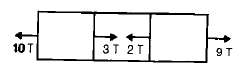Detailed Solution for Test: Solid Mechanics Level - 3 - Question 2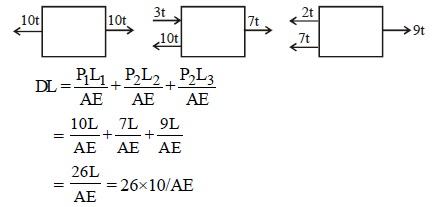Test: Solid Mechanics Level - 3 - Question 3

### The deformation of a bar under its own weight as compared to that when subjected to a direct axial load equal to its own weight will be

Test: Solid Mechanics Level - 3 - Question 4

A slender bar of 100 mm2 cross-section is subjected to loading as shown in the figure below. If the modulus of elasticity is taken as 200 × 109. pa. then the elongation produced in the bar will be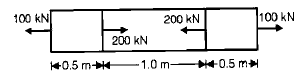Detailed Solution for Test: Solid Mechanics Level - 3 - Question 4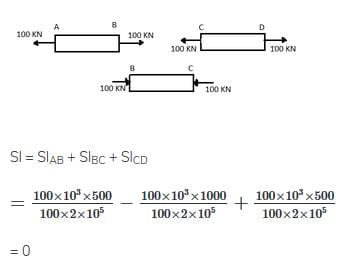Test: Solid Mechanics Level - 3 - Question 5

A straight bar is fixed at edges A and B. its elastic modulus is E and cross-section is A. There is a load P = 120 N acting at C. The reactions at the ends are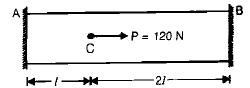Detailed Solution for Test: Solid Mechanics Level - 3 - Question 5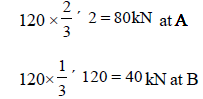Test: Solid Mechanics Level - 3 - Question 6

In a tensile test, near the elastic limit zone

Test: Solid Mechanics Level - 3 - Question 7

If Poisson's ratio of a material is 0.5, then the elastic modulus for the material is

Test: Solid Mechanics Level - 3 - Question 8

The number of independent elastic constants required to express the stress strain relationship for a linearly elastic isotropic material is

Detailed Solution for Test: Solid Mechanics Level - 3 - Question 8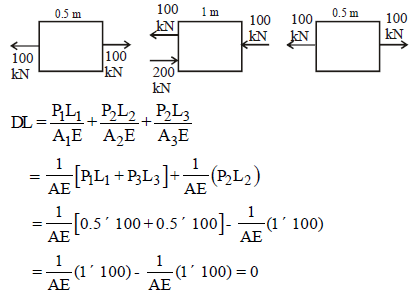Test: Solid Mechanics Level - 3 - Question 9

The number of elastic constants for a completely anisotropic elastic material is

Test: Solid Mechanics Level - 3 - Question 10

For a given material, the modulus of rigidity is 100 GPa and Poisson's ratio is 0.25. The value of modulus of elasticity in GPa is

Detailed Solution for Test: Solid Mechanics Level - 3 - Question 10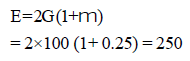Test: Solid Mechanics Level - 3 - Question 11

The volumetric strain in case of a thin cylindrical shell of diameter 'd', thickness 't', subjected to
internal pressure 'P' is

Test: Solid Mechanics Level - 3 - Question 12

The modulus of elasticity for a material is 200 GN/m2 and Poisson's ratio is 0.25. What is the modulus of rigidity ?

Detailed Solution for Test: Solid Mechanics Level - 3 - Question 12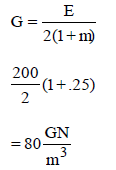Test: Solid Mechanics Level - 3 - Question 13

E, G, K andm represent the elastic modulus, shear modulus, Bulk modulus and poisson's ratio respectively of a linearly elastic. isotropic and homogeneous material. To express the stress-strain relations completely for this material, at least

Test: Solid Mechanics Level - 3 - Question 14

What is the relationship between the linear elastic properties; Young's modulus (E), rigidity modulus (G) and bulk modulus (K) ?

Test: Solid Mechanics Level - 3 - Question 15

A copper rod 400 mm long is pulled in tension to a length of 401.2 mm by applying a tensile stress of 330 MPa. If the deformation is entirely elastic, the Young's Modulus of copper is

Detailed Solution for Test: Solid Mechanics Level - 3 - Question 15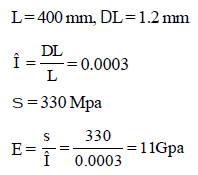Test: Solid Mechanics Level - 3 - Question 16

Match List – I (State of stress) with List -II (kind of loading) and select the correct answer using
the codes given below the lists: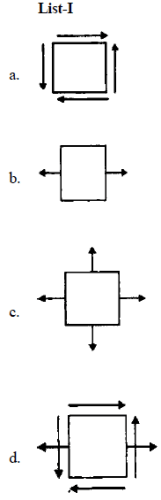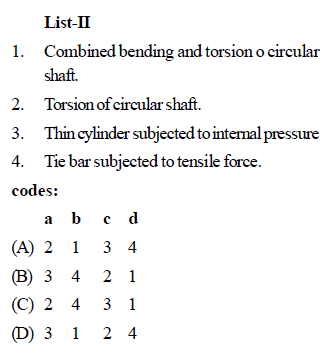Test: Solid Mechanics Level - 3 - Question 17

State of stress at a point in a strained body is shown in figure A. which one of the figure given
below represents correctly the mohr's circle for the state of stress?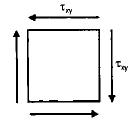Test: Solid Mechanics Level - 3 - Question 18

Plane stress at a point in a body is defined by principal stresses 3s and s. The ratio of the normal stress to the maximum shear stress on the plane of maximum shear stress is

Test: Solid Mechanics Level - 3 - Question 19

Which one of the following Mohr's circles represents the state of pure shear?

Test: Solid Mechanics Level - 3 - Question 20

In a two dimensional problem the state of pure shear at a point is characterized by

Test: Solid Mechanics Level - 3 - Question 21

The unit of work or energy in S.I. units is

Detailed Solution for Test: Solid Mechanics Level - 3 - Question 21

The SI unit of energy/work is the joule (J), named for English physicist James Prescott Joule (1818 - 1889).

Test: Solid Mechanics Level - 3 - Question 22

A circular shaft subjected to twisting moment results in maximum shear stress of 60 MPa. Then the maximum compressive stress in the material is

Test: Solid Mechanics Level - 3 - Question 23

Consider a two dimensional state of stress given for an element as shown in the diagram given below: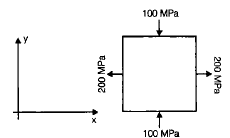What are the coordinates of the centre of Mohr's circle

Detailed Solution for Test: Solid Mechanics Level - 3 - Question 23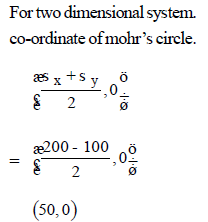Test: Solid Mechanics Level - 3 - Question 24

At a point in two-dimensional stress systems x =100 N/mm2,sy =txy= 400 N/mm2. what drawn with a scale of 1cm = 100 N/mm2.

Test: Solid Mechanics Level - 3 - Question 25

A body is subjected to a pure tensile stress of 100 units. What is the maximum shear produced in the body at some oblique plane due to the above?

## Civil Engineering SSC JE (Technical)

2 videos|111 docs|50 tests
 Use Code STAYHOME200 and get INR 200 additional OFF Use Coupon Code
Information about Test: Solid Mechanics Level - 3 Page
In this test you can find the Exam questions for Test: Solid Mechanics Level - 3 solved & explained in the simplest way possible. Besides giving Questions and answers for Test: Solid Mechanics Level - 3, EduRev gives you an ample number of Online tests for practice

## Civil Engineering SSC JE (Technical)

2 videos|111 docs|50 tests